# Const Keyword in C++ Programming

## Const Keyword in C++

‘const’ keyword stands for constant. In C++ it is used to make some values constant throughout the program. If we make an artifact of a C++ program as constant then its value cannot be changed during the program execution.

In C++, const keyword can be used in a number of ways as discussed below.

Constant Variables

While declaring a variable as const it must be initialized as well. Once declared as const then we cannot change its value later on as shown in the example below.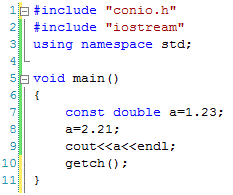We can see that a variable ‘a’ is declared as const at line 7. It is initialized as well with a value 1.23 at the same time. If we try to change its value later on (as at line 8), then C++ compiler will raise an error as below.

error C3892: ‘a’ : you cannot assign to a variable that is const

Using const with Pointers

We can use ‘const’ keyword with pointers too. It can be used in two possible ways:

• We can make a pointer constant at time of declaration.
• We can make the value constant to what the pointer is pointing to.

Constant Pointers

Just like constant variable, the value of constant pointer is initialized at the time of declaration and once declared then we can change the pointer variable. We can also say that if we want that a pointer variable must always point to the same variable then we can make it constant as shown in the example given below.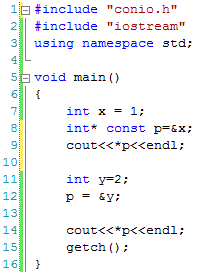We can see that a constant pointer variable ‘p’ is declared at line 8 that points to variable x. As the pointer variable ‘p’ is declared as const so it will always point to variable x. At line 12, we tried to change the value of pointer variable ‘p’ which will lead to a compilation error as below. If the pointer variable was not declared as const then the same code will execute without any error as one pointer variable can point to more than variables at different times.

error C3892: ‘p’ : you cannot assign to a variable that is const

Pointer to Constant Variables

A pointer to const means that we can access the value of the variable through pointer variable but cannot change the value of the pointed variable through the pointer variable as explained in the following example.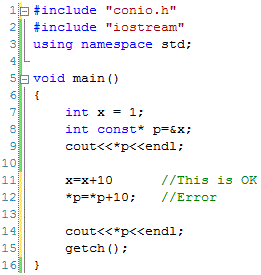The pointer to constant variable is declared at line 8. It is important to note that the variable to what the pointer is pointing to is not constant itself which means that its value can be changed itself (line 11) but cannot be changed through the pointer variable (line 12). If we try to change its value through the pointer variable as at line 12, then it will generate a compilation error as below.

error C2297: ‘*’ : illegal, right operand has type ‘const int *’

Constant Function Arguments

We can use the const keyword with the arguments of a function. If an argument is declared as const then the function will not be allowed to change its value. The same is explained in the following code.At line 5, a constant argument ‘a’ is declared which cannot be changed in the function. If tried (as at line 7) then an error will be raised by compiler as given below.

error C3892: ‘a’ : you cannot assign to a variable that is const

Constant Data Members of a Class

The keyword ‘const’ can also be used with the data members of a class. If we define a const data member of a class then we must have to initialize that data member through the constructor of the class. The value of const data members cannot be changed through the object of the variable as shown in the code below.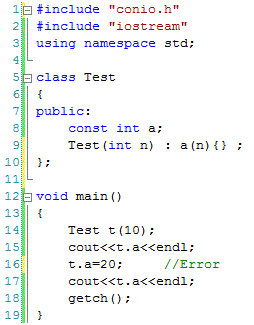In the above code a const data member of a class is declared at line 8 and initialized by the constructor at line 9. This data member can be accessed by the object of the class but cannot be modified. For example at line 15, the value of the const data member is accessed by the object of the class but when tried to change the value of const data member at line 16 then the compiler will not allow it and generates an error.

Constant Objects of a Class

If we declare an object of class as const then the values of data members cannot be changed later on. If we try to change the values of data members then compiler will generate an error as shown in the following code.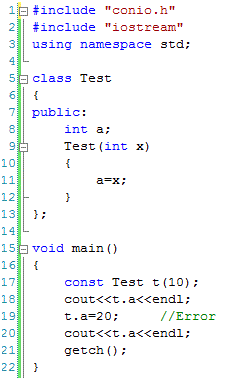In the above code a const object is declared at line 17. Once declared as const object, we cannot change the values of its data members. If we try to change the value of any data member then compiler will not allow us to do so (as shown at line 19 above).